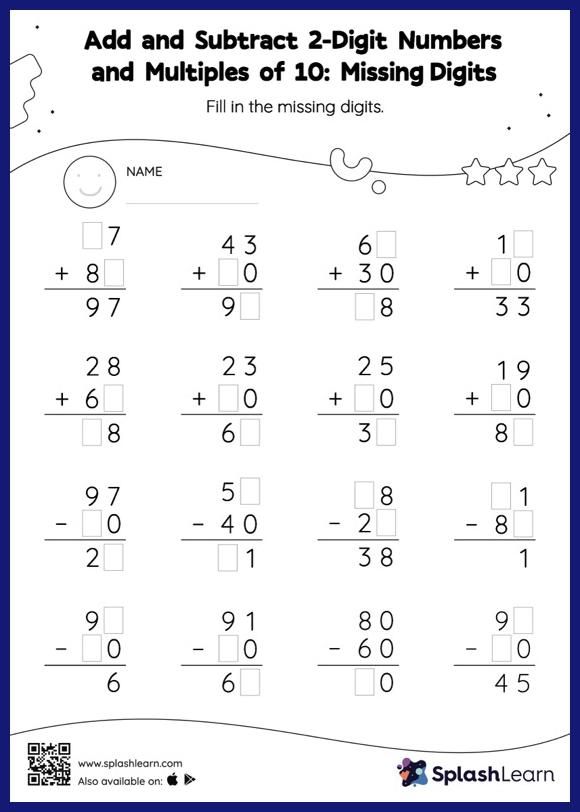# Add and Subtract 2-Digit Numbers and Multiples of 10: Missing Digits Worksheet

Home > Add and Subtract 2-Digit Numbers and Multiples of 10: Missing DigitsStudents demonstrate their problem-solving ability as they work on this add and subtract 2-digit numbers and multiples of 10 worksheet. In add and subtract 2-digit numbers and multiples of 10 worksheet, students must find the missing number using the count-on or count back method or the relationship between addition and subtraction. This worksheet is about practicing the column method in which numbers are written one on top of another. In this method, students use their place value understanding to solve the problems.